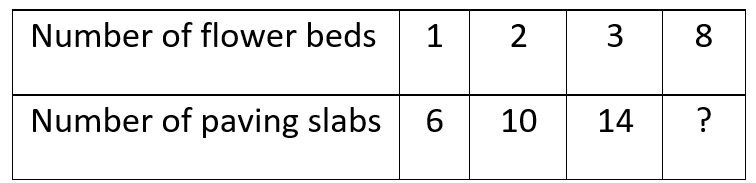General
General
Easy

Question

# The table shows the number of different flower beds formed by a different number of paving slabs.The number of paving slabs that will be needed for 8 flower beds is

## 26    30    34    38Hint:

## The correct answer is: 34

### If we observe the table, we will see that the number of paving slabs is increasing by 4 with increasing number of flower beds.Using this rule, we will find the paving slabs needed for 8 flowers.The paving slabs in one flower = 6The paving slabs in two flowers = 6 + 4 = 10The paving slabs in three flowers = 10 + 4 = 14The paving slabs in four flowers = 14 + 4 = 18The paving slabs in five flowers = 18 + 4 = 22The paving slabs in six flowers = 22 + 4 = 26The paving slabs in seven flowers = 26 + 4 =30The paving slabs in eight flowers = 30 + 4 = 34Therefore, the number of paving slabs needed to make for 8 flowers.

For such questions, we need to find the rule used to write the pattern.

### Related Questions to study#### With Turito Foundation.#### Get an Expert Advice From Turito.